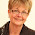## Tuesday, March 4, 2014

### How much fabric do I need for a path?

Thank you for all the get well wishes. I'm feeling a little better but feeling terribly guilty about my silence so I thought I would do a quick post.

A reader asked me how much fabric she would need if she wanted to add single path between her hexagons. The answer of course depends upon a number of factors such as the size of the hexagons and the size of the quilt.

To figure out how much fabric is required you would need to know the number of hexagons form the path. You don't need an exact number; and approximate number is good enough. The simplest way to do this calculation is to diagram one quarter of your quilt on design  paper. Hexagon design sheets can be downloaded for free from Print Free Graph Paper and also from Paper Pieces. In the picture shown below I've sketched out one quarter of a quilt. The "flowers" are made of a single round of 1" hexagons around a center hexagon. I coloured the horizontal rows above and below each set of hexagons.

I count the number of hexagons in each of these rows and the number is 17. There are 5 hexagons (shown as white) that connect the rosettes. I note these numbers down the side of my diagram.

The sum is 105 hexagons for the path. Since the diagram is one quarter of the quilt I multiply this number by four for a total of 420 hexagons. I might need a few more or  few less but this number is close enough to calculate fabric requirements.

The hexagons will be cut from strips of fabric. For 1" hexagons I cut strips that are 2 1/2 inches. You can read more about cutting hexagons from strips of fabric in my post on February 25, 2014.  If you cut 2 1/2" squares from your strip you will end up with 16 to 18 hexagons depending on the width of your fabric. So measure your strip and divide it by 2 1/2" to determine the number of hexagons each strip will yield. Let's assume that we will get 18 hexagons per strip. We know we need 420 hexagons for the path. To determine the number of strips to cut we simply divide 420 by 18. The answer is 23.33333 so we just round that up to 24 strips. The last step to calculate the amount of fabric require is to multiply the number of strips, 24, by the width of the strips 2 1/2". The result is 60" which is 1.7 yards or 1.6 meters! I like to have a little extra just in case so I would round it up to 2 yards/meters. It is that simple!

To recap the formula is:
(Number of Hexagons/Number of Hexagons per strip)*size of strip = number of inches required

so for 1" hexagons in my example the formula is

(420/18)*2.5" = 60"

Do I always calculate the amount of fabric I will need for a path? Usually I don't. If I run out of fabric I simply add a similar fabric to the mix and I think it makes for a more interesting quilt.

Until I post again, stay healthy and happy sewing!
Karen H

1.Wonderful! I don't have a mathematical brain so to me that is magic. Thanks so much - will be very useful. Sorry to hear you have not been well.
Hilda

2.I'd be struggling through that on a good day, let only, not feeling well. Thanks for simplifying things.....again.

3.Thanks for calculating for us the fabrics we may need for a path ! ! ! You're very precious to us!
4.5.6.